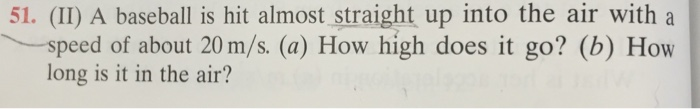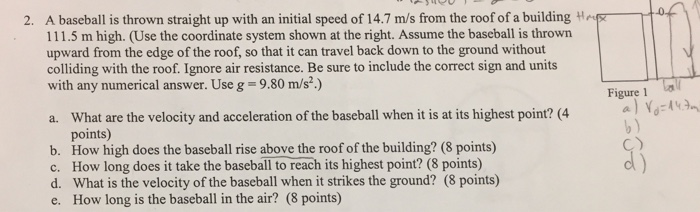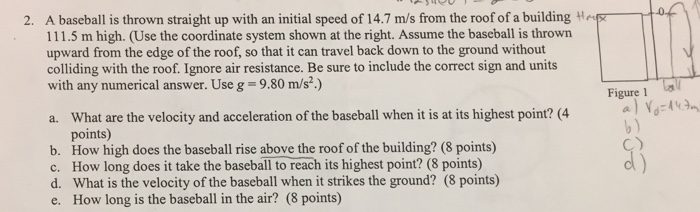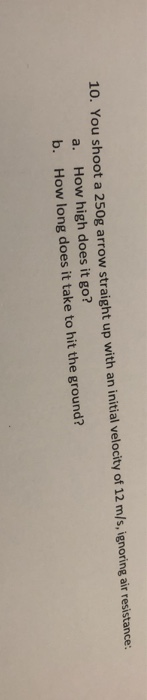# (II) A baseball is hit almost straight up into the air with a speed of about...(II) A baseball is hit almost straight up into the air with a speed of about 20 m/s. (a) How high does it go? (b) How long is it in the air?

apply the formula for Max height H = u^2/2g

where u is intial speed = 20 m/s

g is accletation due to gravity

so

H = (20*20)/(2*9.8)

-----------------------------------------------

apply time period of flgiht

t = 2u/g

t = (2*20)/9.8

#### Earn Coin

Coins can be redeemed for fabulous gifts.

Similar Homework Help Questions
• ### A baseball is hit nearly straight up into the air with a speed of 22 m/s....

A baseball is hit nearly straight up into the air with a speed of 22 m/s. (a) How high does it go? m (b) How long is it in the air? s

• ### A baseball is hit nearly straight up into the air with a speed of 24 m/s

A baseball is hit nearly straight up into the air with a speed of 24 m/s. (a) How high does it go? (b) How long is it in the air?

• ### a baseball is hit almost strait up a kangroo jumps strait up to a vertical height...

a baseball is hit almost strait up a kangroo jumps strait up to a vertical height of 1.75 m. how long was it in the air before returning to earth

• ### I throw a ball straight up in the air with a speed of 15 m/s. It...

I throw a ball straight up in the air with a speed of 15 m/s. It leaves my hand at a height of y = 0 m. a) How high will it go? b) How long does it take to return to its initial height?

• ### Please help me 2. A baseball is thrown straight up with an initial speed of 14.7...Please help me 2. A baseball is thrown straight up with an initial speed of 14.7 m/s from the roof of a building Hp 111.5 m high. (Use the coordinate system shown at the right. Assume the baseball is thrown upward from the edge of the roof, so that it can travel back down to the ground without colliding with the roof. Ignore air resistance. Be sure to include the correct sign and units with any numerical answer. Use g...

• ### Standing on the top ledge of a 55 m high building you throw a ball straight up with an initial speed of 45 m/s

Standing on the top ledge of a 55 m high building you throw a ball straight up with an initial speed of 45 m/s. How long to the nearest second, does it take to hit the ground? I got 10 seconds for the first question. How do I go about answering this one? If the building in the previous problem is 48 meters high and you throw the ball up at 30 m/s, how high to the nearest meter does...

• ### Please help me to solve this physics problem 2. A baseball is thrown straight up with...Please help me to solve this physics problem 2. A baseball is thrown straight up with an initial speed of 14.7 m/s from the roof of a building Hp 111.5 m high. (Use the coordinate system shown at the right. Assume the baseball is thrown upward from the edge of the roof, so that it can travel back down to the ground without colliding with the roof. Ignore air resistance. Be sure to include the correct sign and units with...

• ### A stone is thrown straight up with an initial speed of 80 ft/s

A stone is thrown straight up with an initial speed of 80 ft/s. How high does the stone go, and how long does it stay in the air?

• ### 10. You shoot a 250g arrow straight up with an initial velocity of 12 m/s, ignoring...10. You shoot a 250g arrow straight up with an initial velocity of 12 m/s, ignoring air resistance: a. How high does it go? b. How long does it take to hit the ground?

• ### An arrow is shot straight up in the air with speed of 20m/s

An arrow is shot straight up in the air with speed of 20m/s. How long is t in the air? How high will the arrow rise?